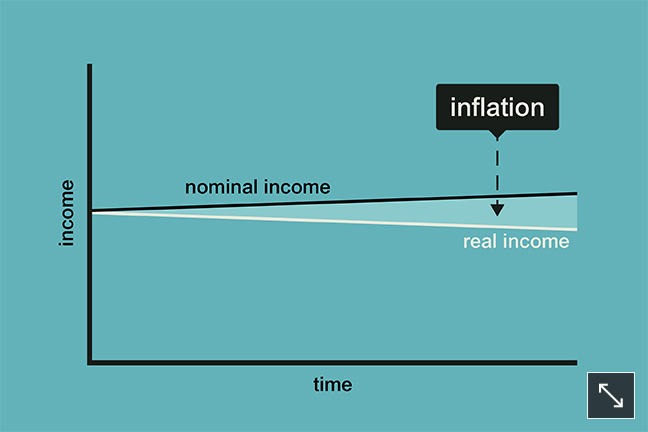2.5

# Measuring real incomesIs your income going up or down in value? (click to expand)

Have a go at measuring change in the real value of incomes.

To do this, download the PDF inflation tables at the bottom of this step. These show the impact of inflation on the purchasing power of incomes.

Let’s say that your monthly gross income today is £1000.

The annual price inflation rate for the next three years is forecast to be 2% (this means that prices will rise by 2% in each of the next three years).

First, you need to know the real value of the £1000 in a year’s time. Go to the column in Table 1 that shows 2% and look at the row for one year. This shows that the real value of your £1000 gross monthly income will be £980.39 in a year’s time.

Now try the calculation again but with an inflation rate of 5%. What’s the outcome?

Now try the calculation again with the original inflation of 2% per annum but for a period of three years. What’s the outcome in three years’ time?

Next, think about the impact on real incomes if there’s an increase in the nominal value of your earnings. Here what you have to do is calculate the difference between the growth of gross income and the rate of price inflation.

For many people in the UK between 2010 and 2015, gross incomes were unchanged or grew at around 1% each year, while prices rose typically at between 2% and 3% each year. So, for many people, a reduction in real value of incomes was experienced in the range of between 1% and 3% each year.

Use Table 2 to see how much loss in value on £1000 results from price inflation exceeding earnings inflation. The top row of the table shows the change in nominal income over a year from a cut of 5% to an increase of 7%. Taking price inflation to be 0.6% over the year, the figures below the percentage change in nominal income show how the real value of income changes.

Now try the exercise using your own circumstances. Can you work out how much your gross income has risen (or fallen) in the past year?

Work out the percentage growth (or decline) and look at the relevant column in Table 2. A deduction of 0.6% is applied to get the real value of incomes, since 0.6% is close to the average rate of price inflation in the UK over the past year.

To calculate the exact figure for you, it is necessary to scale your gross income to the benchmark of £1000 in the table. So, for example, if your gross income is £2000 per month you need to double the values of the real incomes shown.

If you’ve been fortunate enough to see your gross income rise by more than the price inflation rate of 0.6% you can see from Table 2 see how much your real income has increased.

Clearly the relationship between the rate of increase in your gross income and the rate of inflation is central to the determination of whether your standard of living is improving or declining.